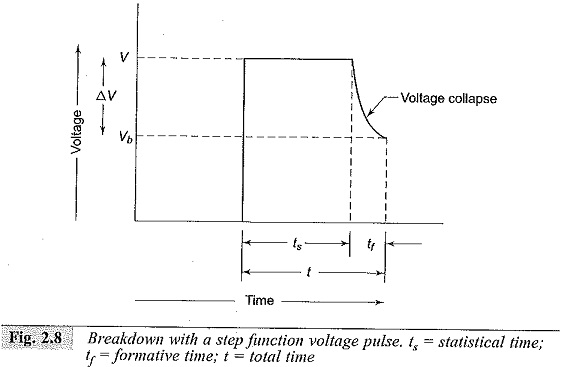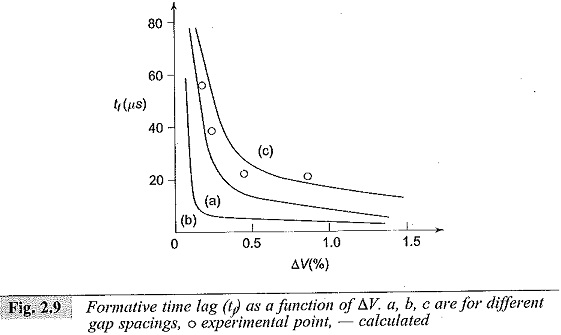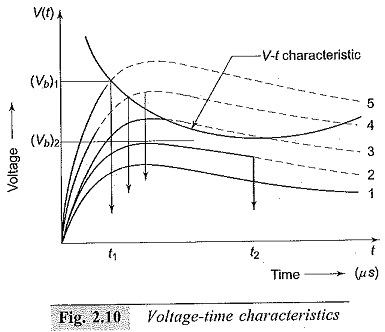## Formative Time Lag:

Formative Time Lag – In the previous section, the mechanism of spark breakdown is considered as a function of ionization processes under uniform field conditions. But in practical engineering designs, the breakdown due to rapidly changing voltages or impulse voltages is of great importance. Actually, there is a time difference between the application of a voltage sufficient to cause breakdown and the occurrence of breakdown itself. This time difference is called the time lag.

The ‘Townsend criterion’ for breakdown is satisfied, only if at least one electron is present in the gap between the electrodes. In the case of applied d.c. or slowly varying (50 Hz a.c.) voltages, there is no difficulty in satisfying this condition. However, with rapidly varying voltages of short duration (≈10-6), the initiatory electron may not be present in the gap, and in the absence of such an electron breakdown cannot occur. The time t which lapses between the application of the voltage sufficient to cause breakdown and the appearance of the initiating electron is called a statistical time lag (ts) of the gap. The appearance of electrons is usually statistically distributed. After the appearance of the electron, a time tt is required for the ionization processes to develop fully to cause the breakdown of the gap, and this time is called the formative time lag (t,). The total time ts+ t= t is called the total time lag as shown in Fig. 2.8.The statistical time lag depends upon the amount of pre-ionization present in the gap. This in turn depends on the size of the gap and the quantity of radiation that produces the primary electrons. The appearance of such electrons is usually statistically distributed. The techniques generally used for irradiating the gaps include ultraviolet radiation, radio active materials and light sources.

The formative time lags depend mostly on the mechanism of the avalanche growth in the gap. In cases where the secondary electrons are produced only due to the bombardment of the cathode by the positive ions, the transit time of the positive ion from the anode to the cathode will predominantly contribute for the formative time lag.

The formative time lag is usually much shorter than the statistical time lag and therefore the statistical time lag can be determined by measuring the total time lag.

### Voltage-time Characteristics:

When an impulse voltage of a value V or higher is applied to a gap,I)reakdown will occur on each voltage application. The time lag will then depend on the rate of rise of the applied voltage and field uniformity. Therefore, for each gap configuration it is possible to construct a volt-time characteristic by applying a number of impulses of increasing magnitude and recording the time lag using an oscilloscope. A representative plot of such a characteristic is shown in Fig. 2.10. In uniform and fairly non-uniform field gaps, the characteristic is sharply defined and rises steeply with increasing rate of rise of the applied voltage.In non­uniform field gaps there will be a large scatter in the results, and the time to breakdown will be less sensitive to the rate of rise of applied voltage. Hence fairly uniform field gaps such as sphere gaps are often used as protective devices against over-voltages in electric power systems. The voltage-time characteristic is an important property of any insulating structure or equipment. It provides a basis for establishing the impulse strength of the insulation as well as for the design of the protective levels against over-voltages in power systems.Time lags are of considerable practical importance. For breakdown to occur the applied voltage V should be greater than the static breakdown voltage Vs as shown in Fig. 2.9. The difference in voltage ΔV = V – Vs is called the overvoltage, and the ratio V/Vs is called the impulse ratio. The variation of tt with overvoltage (ΔV) is shown in Fig. 2.9. The volt-time characteristics of different electrical apparatus are very important in insulation co-ordination.

Scroll to Top